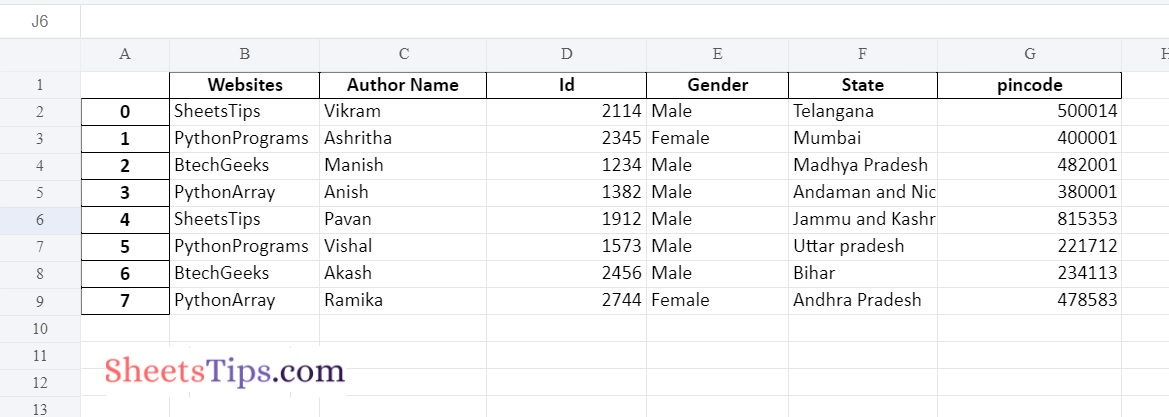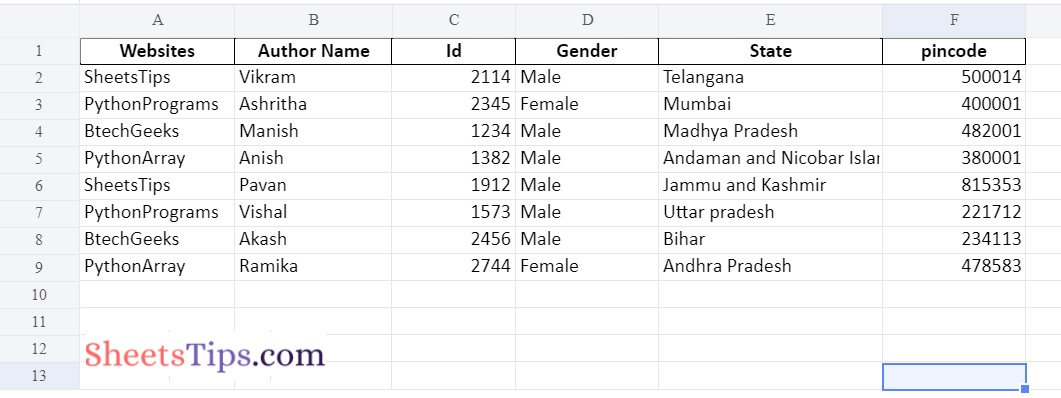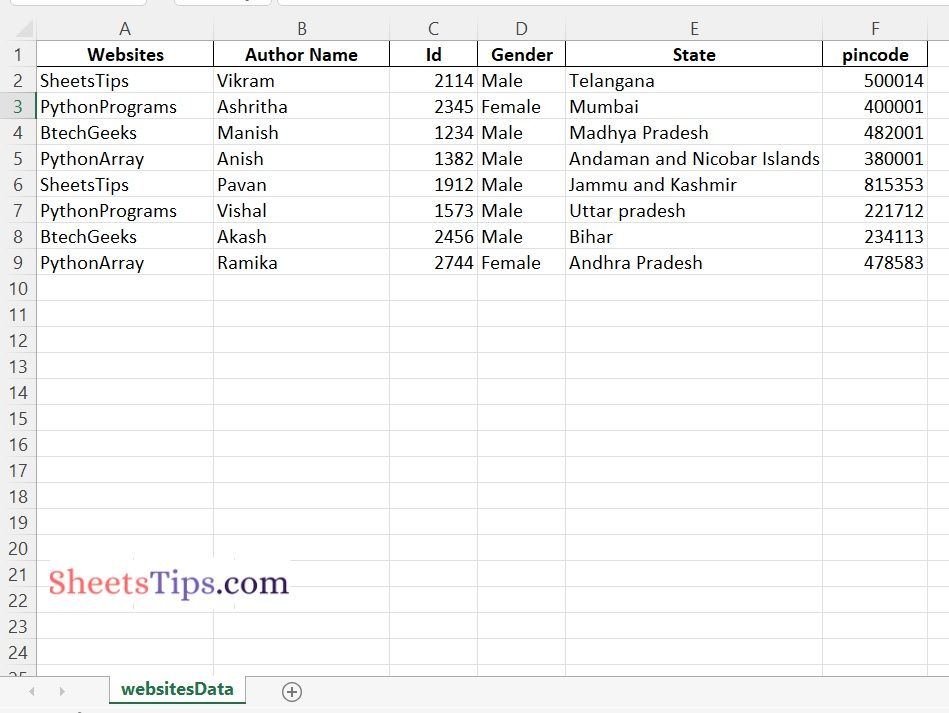# How to Convert JSON to Excel File in Python?

MS Excel is a feature-rich tool that allows you to keep and organise tabular data. It also lets you to store data in several worksheets. Aside from data management, you may also perform sorting, graph charting, mathematical operations, and so on. JSON, on the other hand, is a popular format for storing and transmitting data in key-value pairs. In some circumstances, you may need to dynamically import data from JSON files into Excel workbooks. In line with that, this post will show you how to use Python to convert JSON data to Excel XLSX/XLS.

What do you mean by JSON Array?

JSON (JavaScript Object Notation) is a dictionary-like notation that may be utilized in Python by importing the JSON module. Every record (or row) is preserved as its own dictionary, with the column names serving as the dictionary’s Keys. To make up the whole dataset, all of these records are kept as dictionaries in a nested dictionary. It is saved together with the extension. geeksforgeeks.json

JSON format was actually based on a subset of JavaScript. It is, nevertheless, referred to as a language-independent format, and it is supported by a wide range of programming APIs. In most cases, JSON is used in Ajax Web Application Programming. Over the last few years, the popularity of JSON as an alternative to XML has gradually increased.

While many programs use JSON for data transfer, they may not keep JSON format files on their hard drive. Data is exchanged between computers that are linked via the Internet.

Example:

```{
"Name":"Vikram",
"Branch":"Cse",
"year":2019,
"gpa":[
9.1,
9.5,
9.6,
9.2
]
}```

## Converting JSON to Excel Using Pandas in Python

Below are the methods to convert JSON file to an Excel file:

### Method #1: Using Pandas Library

samplejsonfile.json:

```{
"Websites":[
"SheetsTips",
"PythonPrograms",
"BtechGeeks",
"PythonArray",
"SheetsTips",
"PythonPrograms",
"BtechGeeks",
"PythonArray"
],
"Author Name":[
"Vikram",
"Ashritha",
"Manish",
"Anish",
"Pavan",
"Vishal",
"Akash",
"Ramika"
],
"Id":[
2114,
2345,
1234,
1382,
1912,
1573,
2456,
2744
],
"Gender":[
"Male",
"Female",
"Male",
"Male",
"Male",
"Male",
"Male",
"Female"
],
"State":[
"Telangana",
"Mumbai",
"Andaman and Nicobar Islands",
"Jammu and Kashmir",
"Bihar",
],
"pincode":[
500014,
400001,
482001,
380001,
815353,
221712,
234113,
478583
]
}```

### Step#1: Importing Modules

```# Import json module using the import keyword
import json
# Import pandas module using the import keyword
import pandas as pd```

Here we read the JSON file by specifying the location/path of the json file. Since I am using google colab here, I have uploaded a samplejsonfile.json and directly using the file name.

You can also specify the JSON file path directly, regardless of where it exists on your system.

```# Open JSon file using the open() function by passing the filepath as an argument to it
with open('samplejsonfile.json') as gvn_jsonfile:
# of the json module and store it in a variable

Or we can also use read_json() function of the pandas module to read the JSON File. This is simple as compared to the above one. You can use either of the methods.

```# Pass the json file name as an argument to the read_json() function of the
# pandas module to read the json file and store it in a variable
```

### Step#3: Create a Pandas Dataframe

```# Pass the above json data as an argument to the DataFrame() function of the
# pandas(pd as alias name) module to create a dataframe
# Store it in another variable
datafrme = pd.DataFrame(json_data)```

### Step#4: Converting JSON Data to Excel File and Saving it

Here, we use the to_excel() method of the dataframe object to convert it into an excel file.

```# Apply to_excel() function on the above dataframe to convert the given json data file
# into an excel file and save the output excel with some random name.
datafrme.to_excel('OutputExcelFile.xlsx')```

Now, we can use the dataframe object’s various methods to convert JSON data to other formats such as CSV, SPSS, or STATA, to name a few. To save JSON data to a CSV file, for example, we can use the to_csv method.

### Complete Code

Approach:

• Import json module using the import keyword
• Import pandas module using the import keyword
• Pass the json file name as an argument to the read_json() function of the pandas module to read the json file and store it in a variable
• Pass the above json data as an argument to the DataFrame() function of the pandas(pd as alias name) module to create a dataframe
• Store it in another variable
• Apply to_excel() function on the above dataframe to convert the given json data file
into an excel file and save the output excel with some random name.
• The Exit of the Program.

Below is the implementation:

```# Import json module using the import keyword
import json
# Import pandas module using the import keyword
import pandas as pd

# Pass the json file name as an argument to the read_json() function of the
# pandas module to read the json file and store it in a variable

# Pass the above json data as an argument to the DataFrame() function of the
# pandas(pd as alias name) module to create a dataframe
# Store it in another variable
datafrme = pd.DataFrame(json_data)

# Apply to_excel() function on the above dataframe to convert the given json data file
# into an excel file and save the output excel with some random name.
datafrme.to_excel('OutputExcelFile.xlsx')```

Output:### Converting from JSON TO Excel without Index:

Approach:

• Import JSON module using the import keyword.
• Import pandas module using the import keyword.
• Pass the JSON file name as an argument to the read_json() function of the pandas module to read the JSON file and store it in a variable.
• Pass the above JSON data as an argument to the DataFrame() function of the pandas(pd as alias name) module to create a data frame.
• Store it in another variable.
• Apply to_excel() function on the above data frame to convert the given JSON data file into an excel file and save the output excel with some random name.
• Pass the index as False such that we can remove the indexes from the excel file.
• The Exit of the Program.

Below is the implementation of the above approach:

```# Import json module using the import keyword
import json
# Import pandas module using the import keyword
import pandas as pd

# Pass the json file name as an argument to the read_json() function of the
# pandas module to read the json file and store it in a variable

# Pass the above json data as an argument to the DataFrame() function of the
# pandas(pd as alias name) module to create a dataframe
# Store it in another variable
datafrme = pd.DataFrame(json_data)

# Apply to_excel() function on the above dataframe to convert the given json data file
# into an excel file and save the output excel with some random name.
# Pass the index as False such that we can remove the indexes from the excel file
datafrme.to_excel('OutputExcelFileWithoutIndex.xlsx',index=False)```

Output:Explanation:

```Here by passing index=False as an argument to the to_excel() function we
can remove the indexes from the excel file while saving it```

### Changing the Sheet Name

We can modify the sheet name to any other name we want using the “sheet_name” argument by assigning whatever name we want to it.

The default sheet name is Sheet1, Sheet2,…..

Approach:

• Import JSON module using the import keyword
• Import pandas module using the import keyword
• Pass the JSON file name as an argument to the read_json() function of the pandas module to read the JSON file and store it in a variable.
• Pass the above JSON data as an argument to the DataFrame() function of the pandas(pd as alias name) module to create a data frame.
• Store it in another variable.
• Apply to_excel() function on the above data frame to convert the given JSON data file into an excel file and save the output excel with some random name.
• Pass the index as False such that we can remove the indexes from the excel file.
• Here by using the argument sheet_name we can give any other sheet name we want.
• The Exit of the Program.

Below is the implementation of the above approach:

```# Import json module using the import keyword
import json
# Import pandas module using the import keyword
import pandas as pd

# Pass the json file name as an argument to the read_json() function of the
# pandas module to read the json file and store it in a variable

# Pass the above json data as an argument to the DataFrame() function of the
# pandas(pd as alias name) module to create a dataframe
# Store it in another variable
datafrme = pd.DataFrame(json_data)

# Apply to_excel() function on the above dataframe to convert the given json data file
# into an excel file and save the output excel with some random name.
# Pass the index as False such that we can remove the indexes from the excel file
# Here by using the argument sheet_name we can give any other sheet name we want.
datafrme.to_excel('OutputExcelFileWithoutIndex.xlsx',index=False, sheet_name='websitesData')```

Output:Explanation:

```Here we customized the sheet name to websitesData instead of Sheet1
(which is the default one)```

### Method #2: Using JSON to Excel Converter Package

HTTP Requests to Get Data from the Internet:

HTTP requests are the backbone of the internet. When you navigate to a web page, your browser sends several requests to the server. The server then responds with all of the data required to generate the page, which your browser then renders so you can view it.

The general procedure is as follows: a client (such as a browser or a Python script using Requests) sends data to a URL, and the server located at that URL reads the data, decides what to do with it, and returns a response to the client. Finally, the client can select what to do with the response data.

When making an HTTP request, HTTP methods such as GET and POST define the action you’re trying to do.

The GET method is one of the most often used HTTP methods. The GET method shows that you are attempting to retrieve data from a specific resource.

A Response is a useful object for inspecting the request’s results. Make the same request again, but this time save the response value in a variable so you may examine its attributes and behaviors more closely:

`response=requests.get(url)`

You’ve captured the return value of get(), which is an instance of Response, and saved it in a variable called response in our example. You may now utilize a response to view a lot of information about the outcomes of your GET request.

Of course, there are alternative ways and/or Python packages that can be used to convert JSON to Excel in Python. For example, we may use the json module and one of the packages xlsxwriter or openpyxl. It should be noted, however, that the resulting Python script will be rather more sophisticated. Additionally, there is a Python package created to convert JSON to Excel.

Approach:

• Import Converter from json_excel_converter module using the import keyword.
• Import Writer from xlsx of json_excel_converter module using the import keyword.
• Give the Url of the JSON file and store it in a variable.
• Get the JSON data present from the above URL using the get() function of the requests and store it in a variable
• Call the Converter() Function and Store it in another variable.
• Convert the above JSON response to an excel file using the convert() function and write the result JSON data into some other excel file using the Writer() function.
• The Exit of the Program.

Below is the implementation:

```# Import Converter from json_excel_converter module using the import keyword
from json_excel_converter import Converter
# Import Writer from xlsx of json_excel_converter module using the import keyword
from json_excel_converter.xlsx import Writer

# Give the Url of the json file and store it in a variable
json_url = 'enter-url-here'
# Get the json data present from the above url using the get() function of the
# requets and store it in a variable
json_response = requests.get(url=json_url)
# Call the Converter() Function and Store it in another variable
xl_converter = Converter()
# Convert the above json response to excel file using the convert() function and
# write the result json data into some other excel file using the Writer() function
xl_converter.convert(json_response.json(), Writer(file='OutputExcelFile.xlsx'))```

Sample Output: